# Fit a plane to selected projection centers#

In this tutorial, we will fit a plane to projection centers (PCs) determined from a grid of (5, 5) EBSD patterns from a single crystal silicon wafer. The resulting fitted plane contains one PC per pattern in the dataset from which the grid is obtained. This plane of PCs can be used in subsequent indexing of the dataset. To relate the sample positions of the grid to the PCs, we will test both an affine transformation and a projective transformation, following the work of .

We’ll start by importing necessary libraries

:

%matplotlib inline

import matplotlib.pyplot as plt
import numpy as np
import skimage.filters as skf

from diffsims.crystallography import ReciprocalLatticeVector
import kikuchipy as kp
from orix.crystal_map import PhaseList

plt.rcParams.update(
{
"figure.facecolor": "w",
"figure.dpi": 75,
"figure.figsize": (8, 8),
"font.size": 15,
}
)


Load data lazily (~500 MB) and inspect its shape and step size

:

s = kp.data.si_wafer(allow_download=True, lazy=True)
s

2023-04-07 12:04:36,676 - hyperspy.extensions - INFO - Enabling extension kikuchipy
2023-04-07 12:04:36,746 - numexpr.utils - INFO - NumExpr defaulting to 4 threads.

:

 Title: SignalType: Pattern EBSD 549.32 MiB 54.93 MiB (50, 50|480, 480) (25,10|480,480) 11 Tasks 10 Chunks uint8 numpy.ndarray

Signal Axes

We see that we have (50, 50) patterns of shape (480, 480), which is the full pattern resolution of the NORDIF UF-420 detector the patterns are acquired on. In the axes manager

:

print(s.axes_manager)

<Axes manager, axes: (50, 50|480, 480)>
Name |   size |  index |  offset |   scale |  units
================ | ====== | ====== | ======= | ======= | ======
x |     50 |      0 |       0 |      40 |     um
y |     50 |      0 |       0 |      40 |     um
---------------- | ------ | ------ | ------- | ------- | ------
dx |    480 |      0 |       0 |       1 |     um
dy |    480 |      0 |       0 |       1 |     um


… we see that the nominal step sizes are $$(\Delta x, \Delta y) = (40, 40)$$ $$\mu$$m. The scan spans an area of $$(2 \times 2)$$ $$\mu$$m$$^2$$. The NORDIF UF-420 detector pixel size (unbinned) is about 90 $$\mu$$m. This means that, for example, we expect the PC to shift about 2000 / 90 $$\approx$$ 22 detector pixels when moving horizontally in the scan. To get a better understanding of the expected changes of the PC in the scan, we’ll look at the mean intensity map of the sample.

:

s_mean_nav = s.mean(axis=(2, 3))
s_mean_nav.compute()  # Need this call because data was loaded lazily

[########################################] | 100% Completed | 202.26 ms


Plot it, also annotating the orientation of the scan relative to the sample position in the chamber. We use the plot() method of the EBSD.xmap attribute for this.

:

s.xmap.scan_unit = "um"
fig = s.xmap.plot(
s_mean_nav.data.ravel(),
colorbar=True,
colorbar_label="Mean intensity",
return_figure=True,
)

# Annotate
ax = fig.axes
ax.text(25, 1, "Bottom", va="top", ha="center")
ax.text(25, 49, "Top", va="bottom", ha="center");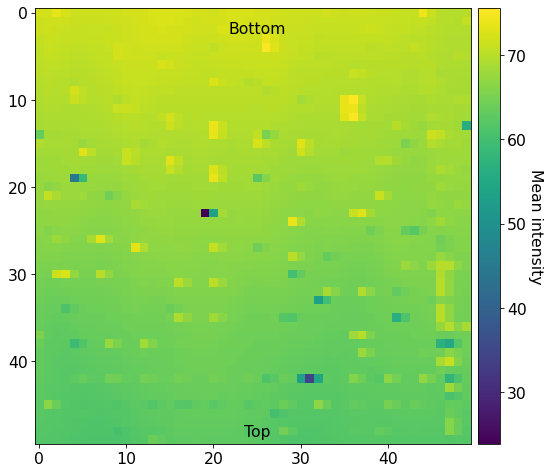The brighter and darker spots come from patterns acquired close to fiducial markers on the Si wafer, while the vertical gradient is a result of the Gaussian intensity distribution varying across the sample. The orientation of the EBSD scan is important to note: To avoid depositing carbon ahead of the scanning beam, the sample is scanned from down the sample upwards, annotated in the map above (“Bottom”, “Top”).

The Bruker PC convention is used internally in kikuchipy. The convention measures (PCx, PCy, PCz) in the following manner, viewing the detector from behind the detector towards the sample:

• PCx from left towards the right

• PCy downwards from the top

• PCz as the shortest distance from the beam-sample interaction position to the detector in fractions of the detector width.

See the reference frames tutorial for more details and conversions between conventions.

Given this definition, let’s annotate on the above map the expected changes to the PC (increasing in the direction of the arrow)

:

annotate_kw = dict(
arrowprops=dict(arrowstyle="-|>", lw=2, mutation_scale=15)
)
xys = [(35, 25), (40, 35), (40, 15)]
xy_texts = [(49, 25), (40, 49), (40, 1)]
has = ["right", "center", "center"]
vas = ["center", "bottom", "top"]
labels = ["PCx", "PCy", "PCz"]
for xy, xytext, ha, va, label in zip(xys, xy_texts, has, vas, labels):
ax.annotate(label, xy=xy, xytext=xytext, ha=ha, va=va, **annotate_kw)

fig  # Show figure again with new annotations

: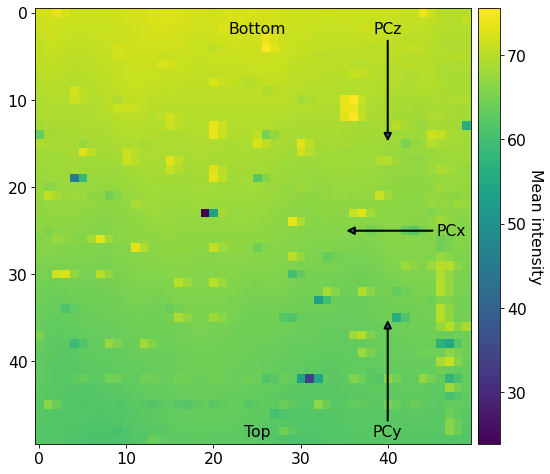Our goal is to fit a plane of (PCx, PCy, PCz) to this (50, 50) map with these variations. To this end, we will perform the following actions:

1. Extract an evenly spaced grid of patterns from the full dataset

2. Get initial guesses of the PC for each grid pattern with Hough indexing (from PyEBSDIndex)

3. Get initial guesses of the orientation for each grid pattern using the PCs with Hough indexing

4. Refine the orientations and PCs simultaneously by matching experimental to dynamically simulated patterns (simulated with EMsoft)

5. Fit a plane to the refined PCs

## Extract a grid of patterns#

Before we extract the grid of patterns and prepare these for indexing by background correction, we obtain a binary mask to remove parts of the pattern without information (typically the corners). We make the mask via thresholding, and find the threshold value by using the minimum threshold algorithm implemented in scikit-image; note that they have other thresholding algorithms that might work better for other datasets.

:

s_mean = s.mean((0, 1))  # (480, 480)
mean_data = s_mean.data.compute()

:

# Threshold
threshold = skf.threshold_minimum(mean_data)

# Extract center pattern for plotting
p0 = s.inav[25, 25].data

fig, axes = plt.subplots(ncols=3, figsize=(12, 4))
for ax, arr, title in zip(
axes,
):
ax.imshow(arr, cmap="gray")
ax.axis("off")
ax.set_title(title)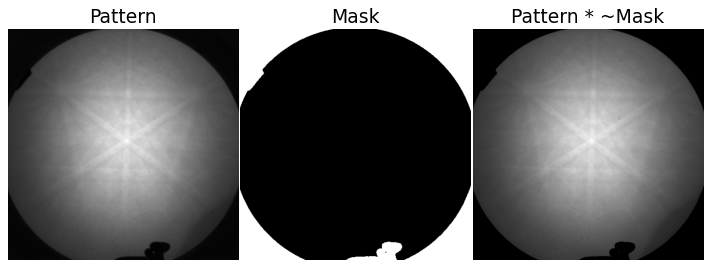The mask efficiently removes the parts of the pattern which contains no information of interest.

Extract the grid using EBSD.extract_grid()

:

grid_shape = (5, 5)
s_grid, idx = s.extract_grid(grid_shape, return_indices=True)

sig_shape_grid = s_grid.axes_manager.signal_shape[::-1]

s_grid.compute()

s_grid

[########################################] | 100% Completed | 102.28 ms

:

<EBSD, title: Pattern, dimensions: (5, 5|480, 480)>


Let’s plot the grid positions on the navigation map

:

kp.draw.plot_pattern_positions_in_map(
rc=idx.reshape(2, -1).T,
roi_shape=(50, 50),
roi_image=s_mean_nav.data,
)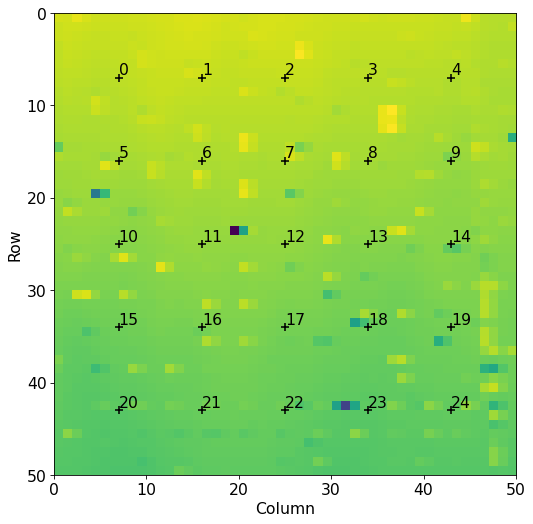We know that all patterns are of silicon. To get a description of silicon, we could create a Phase manually. However, we will later on use a dynamically simulated EBSD master pattern of silicon (created with EMsoft), which is loaded with a Phase. We will use this in the remaining analysis.

:

mp = kp.data.ebsd_master_pattern(
)
mp

:

<EBSDMasterPattern, title: si_mc_mp_20kv, dimensions: (|1001, 1001)>


Extract the phase and change lattice constant unit from nm to Ångström

:

phase = mp.phase

lat = phase.structure.lattice
lat.setLatPar(lat.a * 10, lat.b * 10, lat.c * 10)

print(phase)
print(phase.structure)

<name: si. space group: Fd-3m. point group: m-3m. proper point group: 432. color: tab:blue>
lattice=Lattice(a=5.4307, b=5.4307, c=5.4307, alpha=90, beta=90, gamma=90)
14   0.000000 0.000000 0.000000 1.0000


## Initial guess of PC using Hough indexing#

We will estimate PCs and do Hough indexing of the grid of patterns using PyEBSDIndex. See the Hough indexing tutorial for more details on the Hough indexing related commands below.

Note

PyEBSDIndex is an optional dependency of kikuchipy, and can be installed with both pip and conda (from conda-forge). To install PyEBSDIndex, see their installation instructions.

PyEBSDIndex supports indexing face centered and body centered cubic (FCC and BCC) materials.

Prepare the patterns for indexing by background removal and setting the masked out values to 0

:

s_grid.remove_static_background()
s_grid.remove_dynamic_background()

[########################################] | 100% Completed | 101.33 ms
[########################################] | 100% Completed | 202.86 ms


Get an initial EBSD detector to store PC values in

:

det_grid = s_grid.detector.deepcopy()

print(det_grid)
print(det_grid.sample_tilt)

EBSDDetector (480, 480), px_size 1 um, binning 1, tilt 0.0, azimuthal 0.0, pc (0.5, 0.5, 0.5)
70.0


We need an EBSDIndexer to use PyEBSDIndex. We can obtain an indexer by passing a PhaseList to EBSDDetector.get_indexer()

:

phase_list = PhaseList(phase)
phase_list

:

Id  Name  Space group  Point group  Proper point group     Color
0    si        Fd-3m         m-3m                 432  tab:blue

:

indexer = det_grid.get_indexer(phase_list, nBands=6)
indexer.phaselist

:

['FCC']


We estimate the PC of each pattern with Hough indexing using EBSD.hough_indexing_optimize_pc(), and plot both the mean and standard deviation of the resulting PCs. (We will “overwrite” the existing detector variable in memory.)

:

det_grid = s_grid.hough_indexing_optimize_pc(
pc0=[0.52, 0.16, 0.50],  # Initial guess based on previous experiments
indexer=indexer,
batch=True,
)

print(det_grid.pc_flattened.mean(axis=0))
print(det_grid.pc_flattened.std(0))

[0.51965585 0.15874812 0.49179152]
[0.02239026 0.01748041 0.01067665]


Plot the PCs

:

det_grid.plot_pc("map")
det_grid.plot_pc("scatter", annotate=True)Looking at the maps:

• PCx increases towards the left, as expected.

• PCy increases upwards, as expected.

• PCz seems to increase downwards, as expected, although there are some patterns, especially the 0th, which do not follow this trend.

Looking at the scatter plots:

• We recognize the regular (5, 5) grid in the (PCx, PCy) plot, although some patterns do not align as well as the others (e.g. 0th).

• There is an inverse relation between PCy and PCz: as PCy decreases, PCz increases. This is as expected. However, there is a significant spread in each row of points (5-9, 10-14, etc.).

These initial guesses seem OK, and we could perhaps fit a plane to these values already. But, we can get a better fit by refining the PC values using pattern matching. To that end, we obtain initial guesses for the orientations via Hough indexing with EBSD.hough_indexing(). We get a new indexer with the optimized PCs from above and then index

:

indexer = det_grid.get_indexer(phase_list, nBands=6)

:

xmap_grid = s_grid.hough_indexing(
phase_list=phase_list, indexer=indexer, verbose=2
)

Hough indexing with PyEBSDIndex information:
PyOpenCL: True
Projection center (Bruker, mean): (0.5197, 0.1587, 0.4918)
Indexing 25 pattern(s) in 1 chunk(s)
Convolution Time: 0.0037544520000665216
Peak ID Time: 0.001710380999611516
Band Label Time: 0.0670934159998069
Total Band Find Time: 0.11659346299984463Band Vote Time:  0.03200899400007984
Indexing speed: 74.46543 patterns/s


Check the average pattern fit and confidence metric

:

fig, axes = plt.subplots(ncols=2, figsize=(10, 3.5))
for ax, to_plot, label in zip(
axes, ["fit", "cm"], ["Pattern fit [deg]", "Confidence metric"]
):
im = ax.imshow(xmap_grid.get_map_data(to_plot))
ax.axis("off")We see that the upper left (0th) pattern has a high pattern fit and low confidence metric, which is in line with the PC values of that pattern being an outlier in the scatter plots of (PCx, PCz) and (PCz, PCy) above.

Let us refine these initial guesses of orientations and PCs using the loaded dynamically simulated master pattern.

## Refine PCs using pattern matching#

Let’s look at the dynamical simulation (which is in the square Lambert projection, required for use in dictionary indexing and refinement in kikuchipy [and EMsoft])

:

mp.plot()Refine orientations and PCs using the Nelder-Mead optimization algorithm as implemented in NLopt. During optimization, orientations are represented as three Euler angles, and are varied within a sufficiently wide trust region of +/- 5$$^{\circ}$$ of the orientation obtained from Hough indexing above. PCs are varied within a trust region of +/- 10% of the detector width (for PCx and PCz) or height (for PCy). Optimization of each pattern stops when the increase in normalized cross-correlation (NCC) score between iterations, obtained by comparing experimental to simulated pattern, is lower than $$10^{-5}$$.

:

xmap_grid_ref, det_grid_ref = s_grid.refine_orientation_projection_center(
xmap=xmap_grid,
detector=det_grid,
master_pattern=mp,
energy=20,
trust_region=[5, 5, 5, 0.1, 0.1, 0.1],
rtol=1e-5,
chunk_kwargs=dict(chunk_shape=1),
)

Refinement information:
Trust region (+/-): [5.  5.  5.  0.1 0.1 0.1]
Relative tolerance: 1e-05
Refining 25 orientation(s) and projection center(s):
[########################################] | 100% Completed | 102.16 s
Refinement speed: 0.24470 patterns/s


Check the mean NCC score, mean PC and the standard deviation of the mean PC

:

print(xmap_grid_ref.scores.mean())
print(det_grid_ref.pc_average)
print(det_grid_ref.pc_flattened.std(0))

0.3338941013813019
[0.51860624 0.15434697 0.48645274]
[0.0230258  0.02025432 0.00758214]


Check the distribution of refined PCs

:

det_grid_ref.plot_pc("map")
det_grid_ref.plot_pc("scatter", annotate=True)The grid is easily recognizable in all three scatter plots now. If there were any outliers, we could exclude them by passing a boolean array when fitting a plane. This array can either be created manually, or we can try to find outliers by robustly fitting a line to the (PCz, PCy) plot. Assuming the sample is perfectly tilted about the detector $$X_d$$, the slope of the fitted line is the estimated tilt angle.

:

xtilt, is_outlier = det_grid_ref.estimate_xtilt(
detect_outliers=True, degrees=True, return_outliers=True
)
print(xtilt)

19.375530028270283We (hopefully) see that 24th pattern (and perhaps also the 3rd) is automatically recognized as an outlier. If not, we can update (or create) the outlier mask manually

:

is_outlier[4, 4] = True


We can now fit a plane to the remaining PCs.

## Fit a plane to the refined PCs#

To fit a plane to the PCs, we must pass an array of all indices in the full map and the indices of the patterns which the PCs were estimated from. In the case of the grid patterns we’ve used here, the map indices were returned from the EBSD.extract_grid().

:

nav_shape = s.axes_manager.navigation_shape[::-1]
map_indices = np.indices(nav_shape)


Using EBSDDetector.fit_pc() we will fit a plane to the PCs using both an affine transformation and a projective transformation. The fit method automatically plots the three scatter plots above and a forth 3D plot, with the experimental PC values shown as black circles and the fitted PCs corresponding to the experimental ones as larger gray circles.

:

det_ref_aff = det_grid_ref.fit_pc(
idx,
map_indices=map_indices,
transformation="affine",
is_outlier=is_outlier,
)
print(det_ref_aff)

# Sample tilt
print(det_ref_aff.sample_tilt)

# Max. deviation between experimental and fitted PC
pc_diff_aff = det_grid_ref.pc - det_ref_aff.pc[tuple(idx)]
print(abs(pc_diff_aff.reshape(-1, 3)).mean(axis=0))

EBSDDetector (480, 480), px_size 1 um, binning 1, tilt 0.0, azimuthal 0, pc (0.519, 0.155, 0.486)
70.90115818660841
[0.00076088 0.00133539 0.00070831]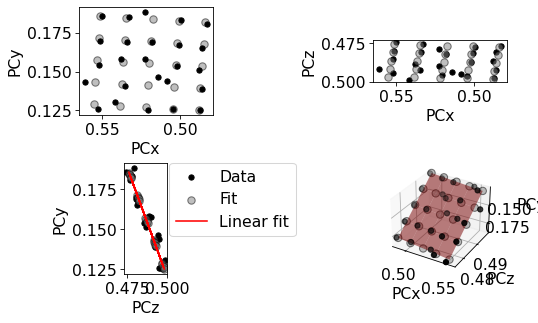:

det_ref_proj = det_grid_ref.fit_pc(
idx,
map_indices=map_indices,
transformation="projective",
is_outlier=is_outlier,
)
print(det_ref_proj)

# Sample tilt
print(det_ref_proj.sample_tilt)

# Max. deviation between experimental and fitted PC
pc_diff_proj = det_grid_ref.pc - det_ref_proj.pc[tuple(idx)]
print(abs(pc_diff_proj.reshape(-1, 3)).mean(axis=0))

EBSDDetector (480, 480), px_size 1 um, binning 1, tilt 0.0, azimuthal 0, pc (0.519, 0.155, 0.486)
70.9959118688575
[0.0006662  0.00096552 0.00075445]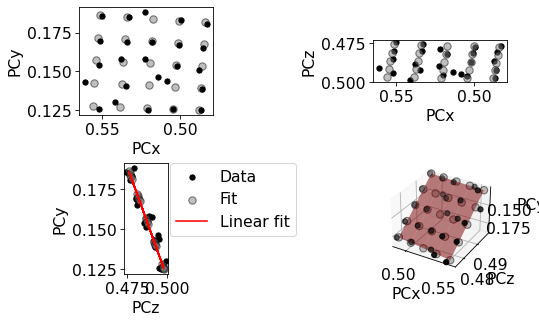We see that the projective plane better fits the experimental PCs than the affine plane. But all-in-all, both planes fit very well.

## Validate fitted PCs#

Finally, we will refine the (already refined) orientations of the grid patterns using the above fitted PCs, and inspect geometrical simulations on top of all patterns.

:

det_ref_proj_grid = det_ref_proj.deepcopy()
det_ref_proj_grid.pc = det_ref_proj_grid.pc[tuple(idx)]

:

xmap_grid_refori = s_grid.refine_orientation(
xmap=xmap_grid_ref,
detector=det_ref_proj_grid,
master_pattern=mp,
energy=20,
trust_region=[5, 5, 5],
rtol=1e-5,
chunk_kwargs=dict(chunk_shape=1),
)

print(xmap_grid_refori.scores.mean())

Refinement information:
Trust region (+/-): [5 5 5]
Relative tolerance: 1e-05
Refining 25 orientation(s):
[########################################] | 100% Completed | 19.35 ss
Refinement speed: 1.29130 patterns/s
0.3339714002609253


To plot geometrical simulations on top of our experimental patterns, we create a KikuchiPatternSimulator using the Si Phase from the master pattern above (see the geometrical EBSD simulations tutorial for more details)

:

rlv = ReciprocalLatticeVector.from_min_dspacing(phase)

# Ensure a complete unit cell (potentially changes the number of atoms)
rlv.sanitise_phase()

rlv.calculate_structure_factor()
structure_factor = abs(rlv.structure_factor)
rlv = rlv[structure_factor > 0.4 * structure_factor.max()]

rlv.print_table()

 h k l      d     |F|_hkl   |F|^2   |F|^2_rel   Mult
1 1 1    3.135    18.4     338.2     100.0      8
2 2 0    1.920    15.0     224.0      66.2      12
4 0 0    1.358     9.2     83.7       24.8      6
3 1 1    1.637     8.5     72.4       21.4      24

:

simulator = kp.simulations.KikuchiPatternSimulator(rlv)


Get one simulation per pattern

:

sim = simulator.on_detector(
det_ref_proj_grid,
xmap_grid_refori.rotations.reshape(*xmap_grid_refori.shape),
)

Finding bands that are in some pattern:
[########################################] | 100% Completed | 104.19 ms
Finding zone axes that are in some pattern:
[########################################] | 100% Completed | 104.42 ms
Calculating detector coordinates for bands and zone axes:
[########################################] | 100% Completed | 101.90 ms


Plot the geometrical simulations on top of the patterns

:

fig, axes = plt.subplots(*grid_shape, figsize=(15, 15))
for i in np.ndindex(grid_shape):
axes[i].imshow((s_grid.data[i]), cmap="gray")
axes[i].axis("off")

lines = sim.as_collections(i)